# scipy.signal.tf2zpk¶

scipy.signal.tf2zpk(b, a)[source]

Return zero, pole, gain (z,p,k) representation from a numerator, denominator representation of a linear filter.

Parameters: b : ndarray Numerator polynomial. a : ndarray Denominator polynomial. z : ndarray Zeros of the transfer function. p : ndarray Poles of the transfer function. k : float System gain.

Notes

If some values of b are too close to 0, they are removed. In that case, a BadCoefficients warning is emitted.

The b and a arrays are interpreted as coefficients for positive, descending powers of the transfer function variable. So the inputs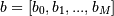and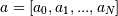can represent an analog filter of the form: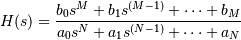or a discrete-time filter of the form: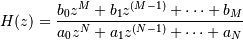This “positive powers” form is found more commonly in controls engineering. If M and N are equal (which is true for all filters generated by the bilinear transform), then this happens to be equivalent to the “negative powers” discrete-time form preferred in DSP: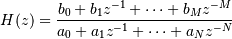Although this is true for common filters, remember that this is not true in the general case. If M and N are not equal, the discrete-time transfer function coefficients must first be converted to the “positive powers” form before finding the poles and zeros.

#### Previous topic

scipy.signal.dstep

#### Next topic

scipy.signal.zpk2tf